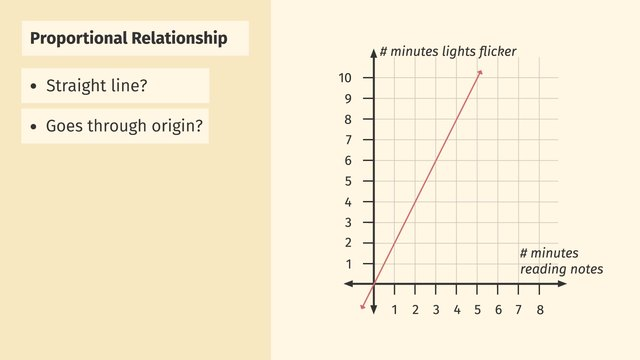# Interpreting Graphs of Proportional RelationshipsRating

Ø 5.0 / 1 ratings

The authorsChris S.

## Basics on the topicInterpreting Graphs of Proportional Relationships

After this lesson, you will be able to identify graphs that represent proportional relationships, find unit rates, and write equations.

The lesson begins by teaching the characteristics of graphs that represent proportional relationships. It leads to finding the slope, k, or constant of proportionality. It concludes with writing equations of proportional relationships.

Learn about the graphs of proportional relationships by helping Detective Egon Spongler uncover the secrets of paranormal activity.

This video includes key concepts, notation, and vocabulary such as the graph of a proportional relationship (a straight line through the origin) and equations representing proportional relationships (y=kx where k is the slope).

Before watching this video, you should already be familiar with the definition of a rate, unit rate, and proportional relationship, as well as identifying these terms from a table that represents relations.

After watching this video, you will be prepared to learn how to plot and interpret points in a coordinate plane, make the connection between a point and the equation of a line containing the point, and identify the equation of a different type of line.

Common Core Standard(s) in focus: 7.RP.A.2 A video intended for math students in the 7th grade Recommended for students who are 12 - 13 years old